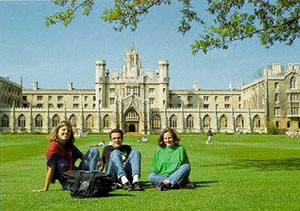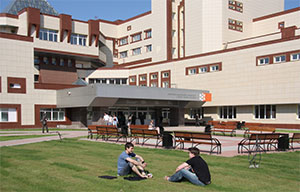ISSN 1026-955X
Vestnik Vysshey Shkoly (Higher School Herald)
The best way to learn all about Higher Education
Languages

=# Cognitive essence of the mathematical picture of the world and the problem of understanding mathematical knowledge

N.V. Mikhailova
80,00 Р

UDC 51:1

https://doi.org/10.20339/AM.02-22.017

Natalia V. Mikhailova, Candidate of Philosophical Sciences, Associate Professor of the Department of Higher Mathematics at Belarusian National Technical University, e-mail: michailova.n@bntu.by

The mathematical picture of the world has general ideas about mathematical objects and their characteristic properties. It is based on some system of initial fundamental basic mathematical concepts and mathematical theories. If the structure of the mathematical picture of the world does not focus on the process of obtaining knowledge, then the philosophy of mathematical education focuses on ways of understanding and substantiating mathematics in terms of the truth of mathematical statements, as well as on ideas about the effectiveness of mathematics in applications.

Key words: mathematical picture of the world, cognitive science, problem of knowledge and understanding.

References

1. Baksansky, O.E. History and current state of cognitive approach. In: Current problems of modern cognitive science. Ivanovo, 2012. Р. 39–43.

2. Testov, V.A. Integration of discreteness and continuity in the formation of the mathematical picture of the world of students. Integration of Education. 2018. Vol. 22. No. 3. P. 480–492.

3. Tselishchev, V.V., Khlebalin, A.V. Proof vs. understanding in mathematical proof. Bulletin of Tomsk State University Journal. Pfilosophy. Sociology. Politology. 2020. No. 54. P. 29–38.

4. Okhlopkov, N.M. Studies of the development patterns of the mathematical picture of the world and the features of the modern mathematics development. Bulletin of M.K. Ammosov North-Eastern Federal University. 2010. Vol. 7. No. 4. P. 139–142.

5. Michailova, N.V. The mathematical world picture as semantic component of university education aspects. Pedagogy and Psychology. Theory and practice. 2018. No. 2. P. 44–46.